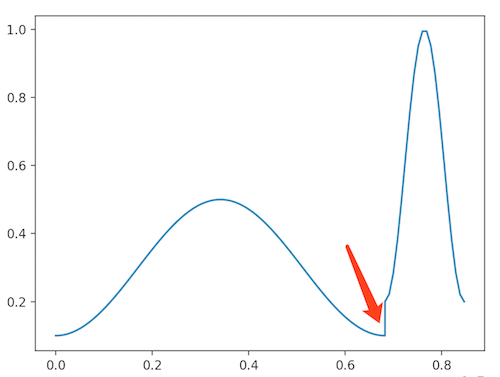# 直播课首页课程排序算法

## 新首页分析## 实现方式

### 时间权重### 代码示例

import time
import datetime
import numpy as np

def calculate_time_weight(time_array):
now = datetime.datetime.now()
now_timestamp = time.time()

past, future, timestamp_array = 0, 0, []
for t in time_array:
timestamp = time.mktime(t.timetuple())
timestamp_array.append(timestamp)
if t > now:
delta = timestamp - now_timestamp
if delta > future:
future = delta
else:
delta = now_timestamp - timestamp
if delta > past:
past = delta

result = []
for t in timestamp_array:
if t > now_timestamp:
rad = 2 * np.pi * ((t-now_timestamp)/future)
result.append(0.4 * np.cos(rad - np.pi) + 0.6)
else:
rad = 2 * np.pi * ((now_timestamp-t)/past)
result.append(0.2 * np.cos(rad - np.pi) + 0.3)
return result

def calculate_sale_weight(sale_array):
max_sale = float(max(sale_array))
return [i/max_sale for i in sale_array]


## 总结

• 老用户占比多，买过的课不会再买，上过的课卖的多，放前面占坑没意义
• 目的主要是拉新，老用户喜欢的所以新用户很大可能是喜欢的这个假设是合理的
• 十个课程，刚好交界地方的顾虑不到